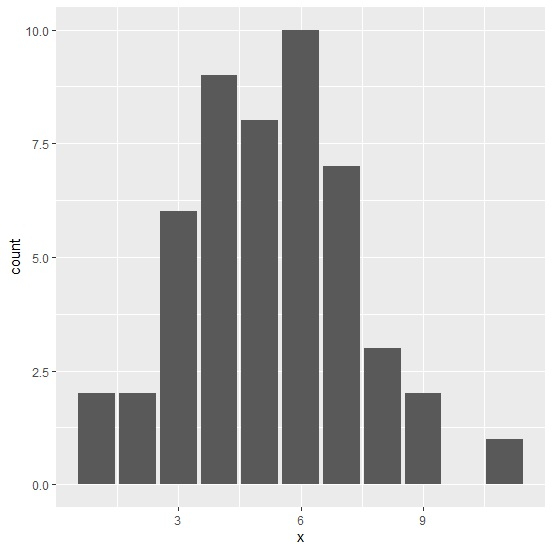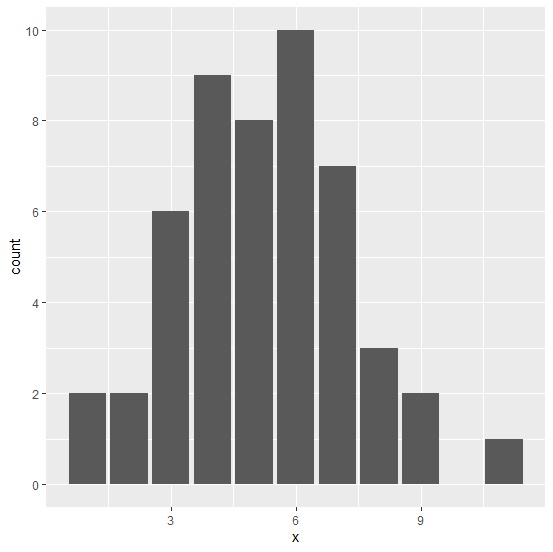# How to change the Y-axis values in a bar plot using ggplot2 in R?

Bar plot is frequently used to analyze the number of times a level of factor variable occurs in a data set and the Y-axis values are crucial to the bar plot. Sometimes these values are not in the form we want, therefore, we want to replace them with the new ones. This can be done with the help of breaks argument of scale_y_continuous function in ggplot2.

## Example

Consider the below data frame −

> set.seed(1)
> x<-rpois(50,5)
> df<-data.frame(x)

> library(ggplot2)

Creating the plot without specifying the Y-axis values −

> ggplot(df,aes(x))+
+ geom_bar()

## OutputPlotting with new Y-axis values −

> ggplot(df,aes(x))+
+ geom_bar()+
+ scale_y_continuous(breaks=c(0,2,4,6,8,10))

## Output